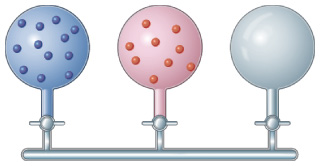# Problem: Consider the apparatus below, which shows gases in two containers and one empty container.Which gas has the greater partial pressure after the stopcocks are opened?

###### FREE Expert Solution

Recall that the partial pressure of a gas (PGas) in a mixture is given by:

$\overline{){{\mathbf{P}}}_{{\mathbf{Gas}}}{\mathbf{=}}{{\mathbf{X}}}_{\mathbf{G}\mathbf{a}\mathbf{s}}{{\mathbf{P}}}_{{\mathbf{Total}}}}\phantom{\rule{0ex}{0ex}}$

where χGas = mole fraction of the gas and Ptotal = total pressure of the gas mixture

The mole fraction of the gas is then given by:###### Problem Details

Consider the apparatus below, which shows gases in two containers and one empty container.Which gas has the greater partial pressure after the stopcocks are opened?